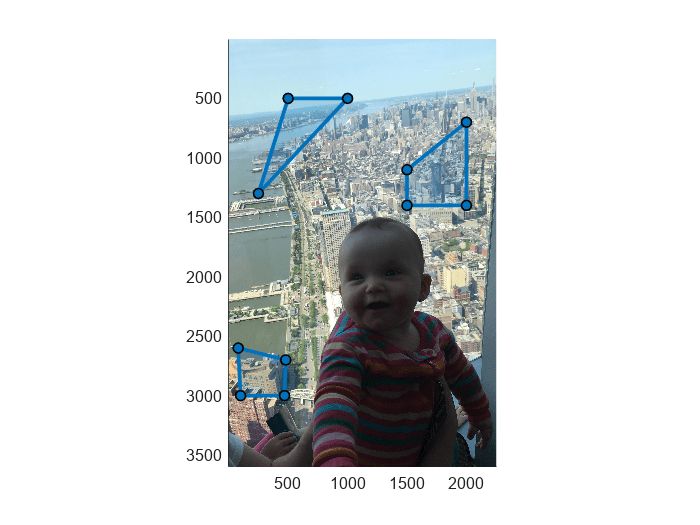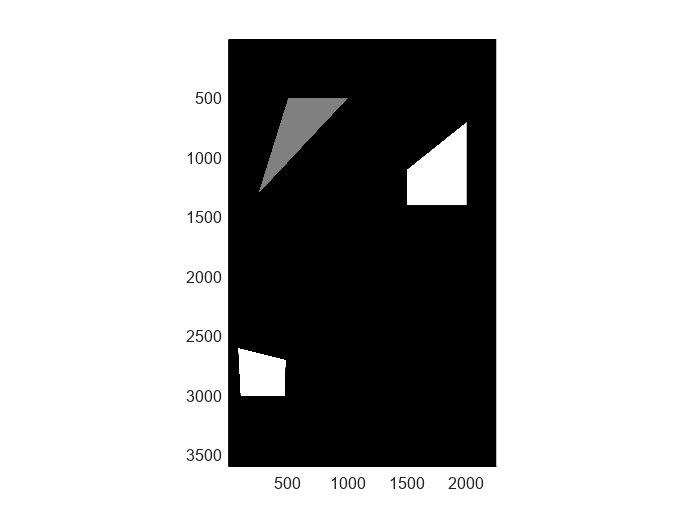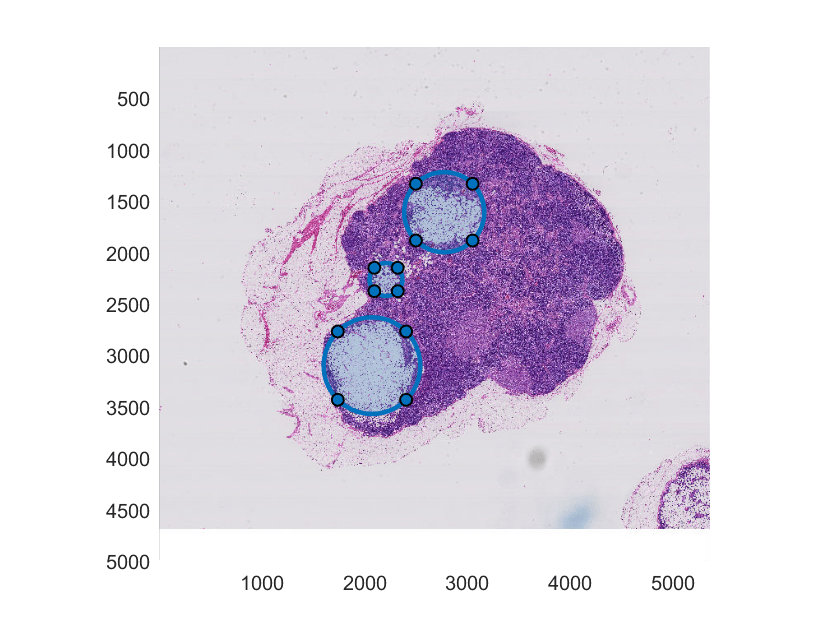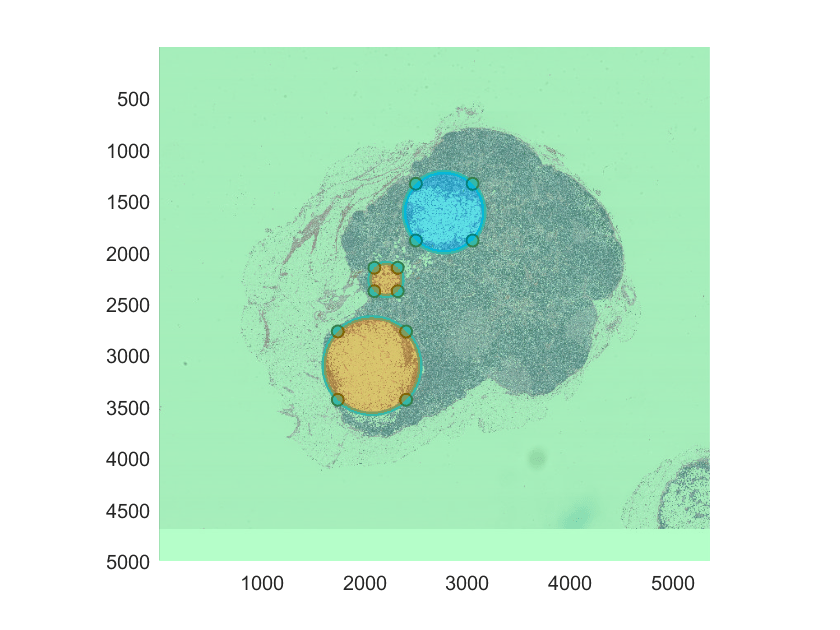polyToBlockedImage

Create labeled blockedImage object from set of ROIs

Description

example

Bout = polyToBlockedImage(ROIpositions,ROILabelIDs,imageSize) creates a numeric, labeled 2-D blocked image Bout of the specified size imageSize from the regions of interest (ROIs) defined in ROIpositions and label IDs defined in ROILabelIDs.

example

Bout = polyToBlockedImage(___,Name=Value) specifies object properties of Bout using name-value arguments in addition to the input arguments from the previous syntax.

Example: polyToBlockedImage(ROIpositions,ROILabelIDs,BlockSize=[512,512]) creates a blocked image with a block size of 512-by-512 pixels.

Examples

collapse all

Create a blocked image.

bim = blockedImage("baby.jpg");

Initialize the ROI position cell array.

numPolygon = 3;
roiPositions = cell(numPolygon,1);

Specify the coordinates of three ROIs in the roiPositions cell array. The first ROI is a triangle, requiring x,y coordinates for three vertices. The other two ROIs are quadrilaterals, requiring x,y coordinates for four vertices.

roiPositions{1} = [500 500; 250 1300; 1000 500];
roiPositions{2} = [1500 1100; 1500 1400; 2000 1400; 2000 700];
roiPositions{3} = [80 2600; 480 2700; 470 3000; 100 3000];

Display the blocked image, and draw the three ROIs on the figure.

bigimageshow(bim);
for id = 1:numPolygon
drawpolygon(Position=roiPositions{id});
endCreate an array for label IDs the same size as the roiPositions cell array.

roilabelID = zeros(numPolygon,1,"uint8");

Specify label ID values that correspond to the order in which you listed the ROIs in roiPositions. The first ROI is a triangle, so label it 1. The other two ROIs are quadrilaterals, so label them 2.

roilabelID(1) = 1;
roilabelID(2) = 2;
roilabelID(3) = 2;

Specify the size of the new labeled blocked image to match the size of the initial blocked image.

imageSize = bim.Size(1:2);

Create a labeled blockedImage object from the ROIs.

L = polyToBlockedImage(roiPositions,roilabelID,imageSize);

Display the labeled blocked image. Use color scaling and color axis limits to visualize the differences between the labels for triangular and quadrilateral ROIs.

bigimageshow(L,CDataMapping="scaled")
caxis([0 2]);Display the labeled blocked image overlaid on the original blocked image.

hbim = bigimageshow(bim);
showlabels(hbim,L)Create a blocked image.

bim = blockedImage("tumor_091R.tif");

Initialize the ROI position cell array.

numPolygon = 3;
roiPositions = cell(numPolygon,1);

Specify the center and radius parameters for three circular ROIs.

center = [2774 1607; 2071 3100; 2208 2262];

Display the blocked image, and use drawcircle to draw the three circular ROIs on the figure. Add the x,y coordinates of the vertices for each ROI to the roiPositions cell array.

hbim = bigimageshow(bim);
for id = 1:numPolygon
roiPositions{id} = hROI.Vertices;
endCreate an array for label IDs the same size as the roiPositions cell array.

roilabelID = zeros(numPolygon,1,"uint8");

Specify label ID values that correspond to the order in which you listed the ROIs in roiPositions. You can assign each ROI a different label, or group multiple ROIs under the same label.

roilabelID(1) = 1;
roilabelID(2) = 2;
roilabelID(3) = 2;

Specify an image size for the new labeled blocked image equal to that of the second resolution level of the initial blocked image, bim.

Create a labeled blockedImage object from the ROIs. Use the WorldStart and WorldEnd name-value arguments to maintain the same world coordinates as the initial blocked image at the specified resolution level.

L = polyToBlockedImage(roiPositions,roilabelID, ...

Display the labeled blocked image overlaid on the original blocked image.

showlabels(hbim,L)Input Arguments

collapse all

Coordinate vectors, specified as a P-element cell array of numeric vectors, where P is the total number of ROIs. Each cell array element is an s-by-2 coordinate vector of the form [x1 y1; … ; xs ys], where s is the total number of vertices for that ROI. Each x,y pair defines a vertex of the ROI. If the ROI shape is not already closed, the polyToBlockedImage function closes the shape automatically. You can specify any number of ROIs.

Data Types: cell

Labels for each ROI, specified as a P-element numeric vector or P-element logical vector, where P is the total number of ROIs.

polyToBlockedImage assigns the value 0 to all background pixels in the output image.

Data Types: single | double | int8 | int16 | int32 | uint8 | uint16 | uint32 | logical

Size of the output labeled blocked image, specified as a numeric vector of positive integers. If you specify more than two dimensions, the polyToBlockedImage function uses only the first two dimensions.

Data Types: single | double | int8 | int16 | int32 | uint8 | uint16 | uint32

Name-Value Arguments

Specify optional pairs of arguments as Name1=Value1,...,NameN=ValueN, where Name is the argument name and Value is the corresponding value. Name-value arguments must appear after other arguments, but the order of the pairs does not matter.

Example: polyToBlockedImage(ROIpositions,ROILabelIDs,imageSize,BlockSize=[512,512]) creates a labeled blocked image with a block size of 512-by-512 pixels.

Read and write interface for the blocked image object, specified as one of these adapter objects.

BINBlocksStore each block as a binary file in a folder
GenericImage Store blocks in a single image
GenericImageBlocksStore each block as an image file in a folder
H5Store blocks in a single HDF5 image
H5BlocksStore each block as an HDF5 file in a folder
InMemoryStore blocks in a variable in main memory
JPEGBlocksStore each block as a JPEG file in a folder
MATBlocksStore each block as a MAT file in a folder
PNGBlocksStore each block as a PNG file in a folder
TIFFStore blocks in a single TIFF file

If OutputLocation is specified, then the function automatically selects an adapter based on the output location. If OutputLocation is not specified, then the default adapter is an InMemory object.

Block size, specified as a numeric vector of positive integers. If you specify more than two dimensions, the polyToBlockedImage function uses only the first two dimensions.

Data Types: double

Wait bar display toggle, specified as a numeric or logical 1 (true) or 0 (false). When set to true, the polyToBlockedImage function displays a wait bar for long-running operations. If you cancel the wait bar, the polyToBlockedImage function returns a partial output, if available.

Data Types: logical

Location to store the output labeled blocked image, specified as a character vector or string scalar. By default, the output blocked image is stored in memory.

Destination TypeImage Format
Folder name (without a file extension)

The polyToBlockedImage function creates the folder and stores blocks of data as files within the folder.

• For numeric image data, polyToBlockedImage stores each block as a binary file using the BINBlocks adapter.

• For categorical and structure image data, polyToBlockedImage stores each block as a MAT file in the folder using the MATBlocks adapter.

File name with TIF or TIFF file extension

The polyToBlockedImage function stores data as a single TIFF image using the TIFF adapter.

The initialValue must be numeric or logical with data type uint8, int8, uint16, int16, uint32, int32, single, double or logical.

File name with H5 file extension

The polyToBlockedImage function stores data as a single HDF5 image using the H5 adapter.

The initialValue must be numeric with data type uint8, int8, uint16, int16, uint32, int32, single, or double.

[]

The blockedImage object stores data as a variable in memory using the InMemory adapter.

To specify a custom adapter for other output formats, use the Adapter property.

World coordinates of the starting edge of the image, specified as a numeric vector. By default, the value is [0.5,0.5].

Data Types: double

World coordinates of the ending edge of the image, specified as a numeric vector. By default, the value is imageSize + 0.5, resulting in pixels that are one unit wide.

Data Types: double

Output Arguments

collapse all

Numeric labeled 2-D blocked image, returned as a blockedImage object.

Tips

• To create a labeled blocked image, Bout, to overlay on an existing blocked image, match imageSize to the size of the existing blocked image at the desired resolution level. If the resolution level of Bout matches the finest resolution level of the existing blocked image, you can use the default values for WorldStart and WorldEnd. To display the overlay at a coarse resolution level, specify WorldStart and WorldEnd to match the world extents of the existing blocked image at the desired resolution level.

• Creating the labeled blocked image at a coarser resolution level decreases the memory required to store the new blocked image, but decreases the smoothness of the ROI edges.

• If a pixel is inside multiple overlapping ROIs, the function assigns the pixel the label corresponding to the overlapping ROI with the lowest index in ROIpositions.